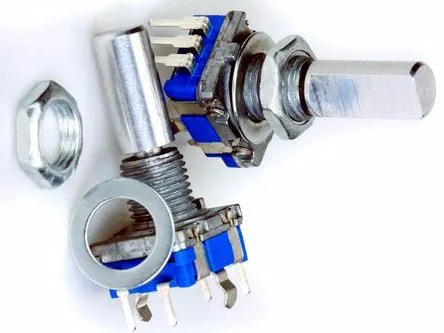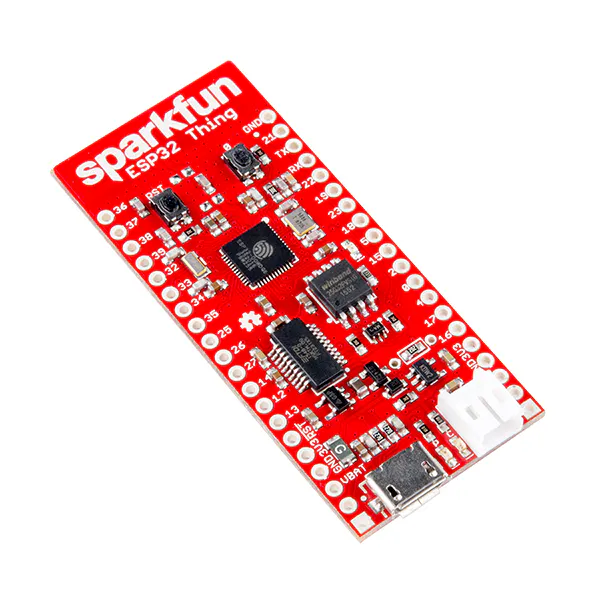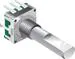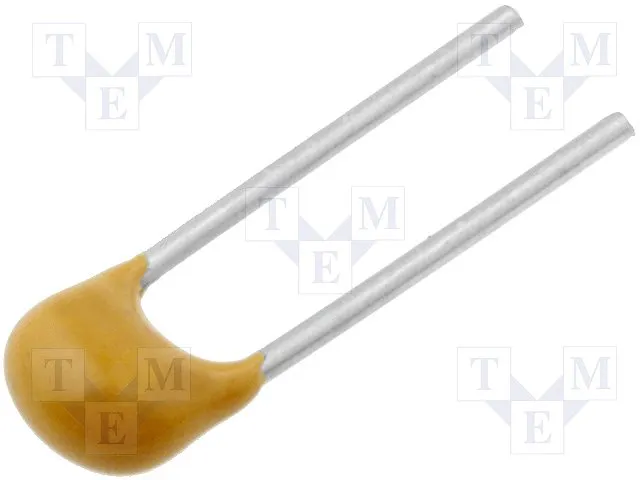# Rotary Quadrature Encoder: Let's Make A Digital Safe...

Build a treasure chest or use an existing storage box, attach a push-button quadrature rotary encoder (endless spinning dial) and lets go!

BeginnerProtip13,198## Things used in this project

### Hardware componentsSparkFun ESP32 Thing Optional, may be any ESP32 device. *Need one of either of these
×1Arduino Nano R3 Optional, any Arduino should work. *Need one of either of these
×1Rotary Encoder with Push-Button https://www.amazon.co.uk/gp/product/B0085I4D5C/
×1
 Knob 6mm shaft, and any knob would do, 3d print yourself something more like a Safe Dial by adapting this: https://www.thingiverse.com/thing:169291
×1×1Jumper wires (generic)
×1Capacitor 100 nF
×13

### Software apps and online servicesArduino IDE

### Hand tools and fabrication machines

 Common Sense Basic electrical knowledge, health and safety of you and others, ask an old person...

## Custom parts and enclosures

### Safe Dial

3d Print this after adjusting to accommodate your switches shaft, or use a pre-built knob.

## Schematics

Fritzing file showing setup

## Code

### esp32 arduino code FIRST ATTEMPT

Arduino
Copy and paste, adjust pin numbers, using pins under 32, or adjust function to be gpio_input_get_high() if you wish to use the top 32 gpio register. -- NEEDS PULL UP RESISTORS OR pinmode(INPUT_PULLUP)
```#define ENC_A 11
#define ENC_B 21

// initially was going to hand pull the required bits from esp32 gpio via
// the 32bit functions gpio_input_get() and gpio_input_get_high() and test
// something like gpio_input_get() & (1<<11 | 1<< 21) == (1<<11 | 1<<21)
// but looking for the docs on the functions I found this line in gpio.h

//#define GPIO_INPUT_GET(gpio_no)     ((gpio_no < 32)? ((gpio_input_get()>>gpio_no)&BIT0) : ((gpio_input_get_high()>>(gpio_no - 32))&BIT0))

// this will involve two calls to the function which is not okay really,
// as the pins may have changed value between calls potentially. One should
// suffice, maybe at the expense of a second variable or additional shifts.
#include <rom/gpio.h>

void setup()
{
/* Setup encoder pins as inputs */
pinMode(ENC_A, INPUT); // OR INPUT_PULLUP
digitalWrite(ENC_A, HIGH);
pinMode(ENC_B, INPUT);
digitalWrite(ENC_B, HIGH);
Serial.begin (115200);
Serial.println("Start");
}

void loop()
{
static uint8_t counter = 0;      //this variable will be changed by encoder input
int8_t tmpdata;
/**/
if( tmpdata ) {
Serial.print("Counter value: ");
Serial.println(counter, DEC);
counter += tmpdata;
}
}

/* returns change in encoder state (-1,0,1) */
{

static int8_t enc_states[] = {0,-1,1,0,1,0,0,-1,-1,0,0,1,0,1,-1,0};
static uint8_t old_AB = 0;
static uint32_t curval = 0;
/**/
old_AB <<= 2;                   //remember previous state
//bit shift old_AB two positions to the left and store.

curval = gpio_input_get();  // returns gpio pin status of pins - SEE DEFINE

//note to self: these curval bits are probably backwards...

old_AB |= ( ( (curval & 1<< ENC_A ) >> ENC_A  | (curval & 1<< ENC_B ) >> (ENC_B - 1) ) & 0x03 );
//add current state and hopefully truncate to 8bit

return ( enc_states[( old_AB & 0x0f )]);
// return the array item that matches the known possible encoder states

// Thanks to kolban in the esp32 channel, who has a great book on everything iot,
// for his initial help at my panic on the esp32 gpio access. long live IRC :)
}
```

### ESP32 WORKING CODE

Arduino
```#define ENC_A 12
#define ENC_B 13

// initially was going to hand pull the required bits from esp32 gpio via
// the 32bit functions gpio_input_get() and gpio_input_get_high() and test
// something like gpio_input_get() & (1<<11 | 1<< 21) == (1<<11 | 1<<21)
// but looking for the docs on the functions I found this line in gpio.h

//#define GPIO_INPUT_GET(gpio_no)     ((gpio_no < 32)? ((gpio_input_get()>>gpio_no)&BIT0) : ((gpio_input_get_high()>>(gpio_no - 32))&BIT0))

// this will involve two calls to the function which is not okay really,
// as the pins may have changed value between calls potentially. One should
// suffice, maybe at the expense of a second variable or additional shifts.
#include <rom/gpio.h>

void setup()
{
/* Setup encoder pins as inputs */
pinMode(ENC_A, INPUT_PULLUP);
digitalWrite(ENC_A, HIGH);
pinMode(ENC_B, INPUT_PULLUP);
digitalWrite(ENC_B, HIGH);
Serial.begin (115200);
delay(10);
delay(10);

//Serial.println("Start");
}

void loop()
{
static uint8_t counter = 0;      //this variable will be changed by encoder input
int8_t tmpdata;
/**/
if( tmpdata ) {
Serial.print("Counter value: ");
Serial.println(counter, DEC);
counter += tmpdata;
}
else{
//   Serial.println("No Change");
}
delay(10);

}

/* returns change in encoder state (-1,0,1) */
{

static int8_t enc_states[] = {0,-1,1,0,1,0,0,-1,-1,0,0,1,0,1,-1,0};
static uint8_t old_AB = 0;
static uint32_t curval = 0;
static uint32_t curtmpA = 0;
static uint32_t curtmpB = 0;
/**/
old_AB <<= 2;                   //remember previous state
//bit shift old_AB two positions to the left and store.

curval = gpio_input_get();  // returns gpio pin status of pins - SEE DEFINE
// Serial.println("curval");
//Serial.println(curval);
//note to self: these curval bits are probably backwards...
curtmpA = (curval & 1<< ENC_A ) >> ENC_A;
//  Serial.println("curtmp A");
//Serial.println(curtmpA);

curtmpB=(curval & 1<< ENC_B ) >> (ENC_B - 1);
//Serial.println(curtmpB);
old_AB |= ( ( curtmpA | curtmpB ) & 0x03 );
//add current state and hopefully truncate to 8bit

return ( enc_states[( old_AB & 0x0f )]);
// return the array item that matches the known possible encoder states

// Thanks to kolban in the esp32 channel, who has a great book on everything iot,
// for his initial help at my panic on the esp32 gpio access. long live IRC :)
}
```

### arduino sketch

Arduino
```#define ENC_PORT PINC
void setup()
{
/* Setup encoder pins as inputs */
pinMode(A0, INPUT);
digitalWrite(A0, HIGH);
pinMode(A1, INPUT);
digitalWrite(A1, HIGH);
Serial.begin (115200);
Serial.println("Start");
}

void loop()
{
static uint8_t counter = 0;      //this variable will be changed by encoder input
int8_t tmpdata;
/**/
if( tmpdata ) {
Serial.print("Counter value: ");
Serial.println(counter, DEC);
counter += tmpdata;
}
}

/* returns change in encoder state (-1,0,1) */
{
static int8_t enc_states[] = {0,-1,1,0,1,0,0,-1,-1,0,0,1,0,1,-1,0};
static uint8_t old_AB = 0;
/**/
old_AB <<= 2;                   //remember previous state
old_AB |= ( ENC_PORT & 0x03 );  //add current state
return ( enc_states[( old_AB & 0x0f )]);
}
```

## Credits

### Tyeth

5 projects • 5 followers
Love solving problems